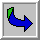SM25S_11834:Thu:1435:219
XXI International Congress of Theoretical and Applied Mechanics
Warsaw, Poland, August 15-21, 2004

A power Flow Mode Theory Based on Inherent Characteristics of Damping Distributions in Systems and Its Applications

 Y. Xiong, J. T. Xing, W. G. Price University of Southampton, Southampton, UK

A generalized power flow mode theory is developed to describe the power-flow behaviour of a dynamical system based on the inherent characteristics of the system?s damping distribution. The eigenvalues and eigenvectors of the damping matrix are defined as the characteristic damping factor and the power flow mode vectors of the system, respectively. These power flow mode vectors are chosen as a set of base-vectors spanning the power flow space and completely describe the power flow of the system. The generalized coordinate of the velocity vector decomposed in the power flow space is defined as the characteristic velocity. The time-averaged power flow is determined from the characteristic damping factor and the characteristic velocity. This demonstrates that for any system with prescribed damping, the power flow of the system is determined if the velocity is derived analytically, numerically or experimentally without requiring force information. Two distinct examples are provided to demonstrate applicability and generality of the theory. This power flow mathematical model allows development of guidelines for the design of dynamic systems based on knowledge of the damping distribution in the system.

Extended Summary (PDF)BACK